# 6. Work and energy

## IntroductionIn a pole vault, the kinetic energy of the initial race is converted into elastic potential energy of the folded pole. As the pole recovers it straight form, this elastic potential energy is transformed into gravitational potential energy. At the instant the pole is in a vertical line, the initial kinetic energy of the run has become gravitational potential energy; the jumper still pushes down the pole, increase its gravitational potential energy even more and going higher. Finally, the jumper releases the pole and falls freely, letting its gravitational potential energy become kinetic energy.

## 6.1. Work and kinetic energy

Newton's second law (equation  4.4)

6.1

where is the resultant of all external forces, leads to a useful relation called the work and kinetic energy theorem. To derive this theorem, consider an infinitesimal vector displacement during an infinitesimal interval of time (Figure  6.1 ).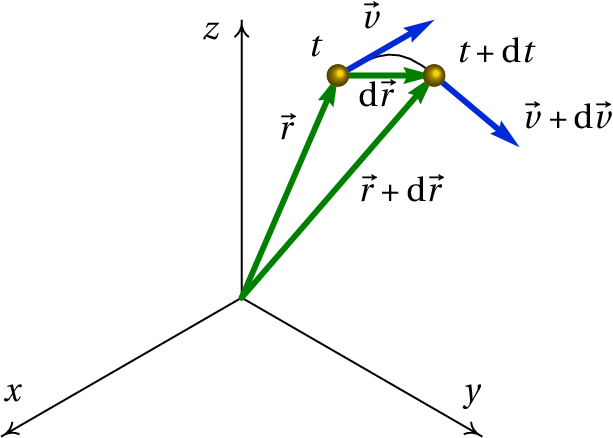Figure 6.1: Position and velocity vectors in an instant t and at a later time t + d t .

In the limit when goes to zero, the vector displacement is in the tangential direction and with magnitude equal to the displacement along the trajectory:

(6.2)

Using this expression and multiplying with scalar product the two sides of equation  6.1 by the infinitesimal displacement, we obtain

(6.3)

The kinematic equation implies that is equal to and hence,

(6.4)

Integrating the two sides of the equation from a position , where the velocity is , to another position where the velocity is , we obtain the work and kinetic energy theorem:

(6.5)

The function of the speed:

(6.6)

Is called kinetic energy and the integral of the tangential component of the force along the trajectory is called work:

(6.7)

The work and kinetic energy theorem states that

The work done by the resultsnt force, along the trajectory, is equal to the increase of the kinetic energy of the particle.

It should be noted that in general the work of a force can be calculated by integrating along any curve, but if this curve is not the trajectory of the particle, the result may not be equal to the increase in kinetic energy. In general, a line integral between two points produces different results ​​for different curves joining these points.

Only the tangential component of the resultant force does work along the trajectory and can change the kinetic energy of the particle. The normal component of the resultant force doesn't do any work and doesn't change the kinetic energy of the particle.

Work and kinetic energy both have units of energy, that is, joules in the International System of Units (1 J = 1 N·m).

In cartesian coordinates, the infinitesimal displacement is,

(6.8)

### Example 6.1

A cannon fires a spherical bullet with radius of 5 cm from the terrace of a building, from the initial position (in meters):

And with initial speed (meters per second):

Determine the maximum height reached by the bullet and the position where the bullet hits the ground (height equal to zero).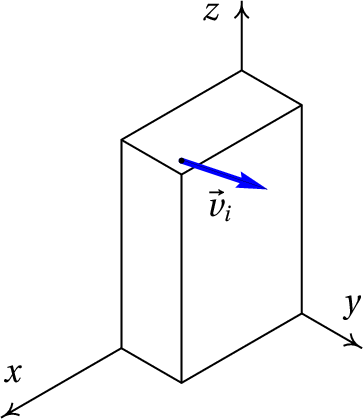Resolution. This is the same problem as example 2.2 that has already been solved in chapter 2, but we will now solve it through work and impulse. A metal bullet has a density approximately 8 times greater than that of water. Under these conditions, the terminal velocity of the bullet is of the order of 132 m/s. The problem will be solved by ignoring the air resistance and in the solution obtained we will be compare the maximum velocity with the terminal velocity. A value of the maximum velocity much smaller than the terminal velocity will confirm that the air resistance is indeed negligible.

In the system of axes of the figure, the weight is written and the impulse it produces from the moment the bullet is released, , and a later instant is,

Equating the impulse to the increase of the quantity of motion and dividing by the mass, we obtain:

(6.9)

Therefore, the and components of the velocity remain constant. The speed will have a minimum value at the instant such that . The minimum value of the speed is then and it corresponds to the point of maximum height.

The work performed by the weight is:

Equating to the variation of the kinetic energy and dividing by the mass,

(6.10)

We can now obtain the maximum height by replacing by the minimum speed

To determine the position where the bullet hits the ground, we the speed with which the bullet hits the ground, substituting in equation 6.10:

And, according to equation 6.9, the square of the speed is:

Where it was taken into account that is positive. During that time, the horizontal displacement is equal to: , since the horizontal component of the velocity is constant. Adding the values ​​of the and components in the initial position, we obtain the position where the bullet hits the ground:

Note that the results are slightly different from those obtained in Example 2.2. In both cases the intermediate results were presented rounding up to 4 significant digits, but all calculations were done using double-precision floating point format (16 significant digits). The difference is that although the time it takes for the bullet to hit the ground appears to be the same in both cases (3.855 s) the stored values ​​in double precision are different because different methods have been used and the numerical error is different in both cases.

The maximum speed, reached when the bullet hits the ground, is 34.55 m/s. Since this value is much smaller than the terminal velocity (132 m/s), the solution obtained ignoring the air resistance will not be too far from the true solution.

The work and kinetic energy theorem contains only a part of the information contained in Newton's second law, since the vector equation 6.1 is actually 3 equations (one for each component) conveniently grouped into vectors. However, it is possible to extract the same three equations from the kinetic energy. Taking into account that:

(6.11)

Then the three cartesian components of equation 6.1 are obtained as follows:

(6.12)

And in an analogous way for the and components. This equation will be generalized to other system of coordinates, different from cartesian, and to non-inertial frames, in chapter 8.

## 6.2. Conservative forces

A force which depends solely on the position is called conservative, if its line integral between two points in the positions and ,

(6.13)

gives the same result along any curve from to .

Therefore, it is possible to choose an arbitrary position and define a function at any position:

(6.14)

notice that this definition implies at the position .

The function cannot be defined when the result of the line integral in 6.14 is not uniquely defined, namely, when the integral gives different results from different paths. The choice of the negative sign in the definition will be explained later. The function , with units of energy, is called the potential energy associated with the conservative force . The advantage of defining potential energies is that is a scalar function, simpler than the vector function , which allows to fully characterize the force. That is, given the potential energy function, it is possible to find the expression of the associated force.

The definition of the potential energy implies that the line integral of the conservative force is the same as:

(6.15)

This result is called the work and potential energy theorem:

The work done between two points by a conservative force is equal to the decrease of the potential energy associated with that force.

Note that the work is equal to the decrease in potential energy, not its increase, due to the use of the negative sign in the definition of the potential energy. It should also be noted that definition 6.14 implies that the potential energy has zero value at the reference position . The effect of changing the reference position is to add or subtract a constant from at all points, but the potential energy differences, , are independent of the position used as reference. The numerical value of the potential energy at a point has no physical meaning; what has significance is the difference in potential energy values ​​at two points.

### Example 6.2

Compute the line integral of the force , from the origin O to the point P in the plane, with coordinates , using the 3 paths indicated in the figure: C1 is the line segment (R with coordinates , ), followed by the straight segment , C2 is the straight segment (Q with coordinates , ), followed by the straight segment and C3 is the straight segment .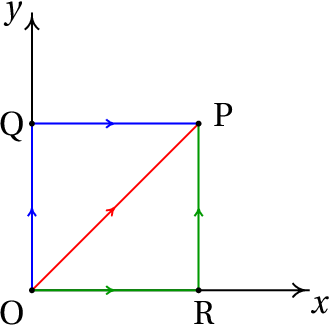Resolution. The vector equation of the line segment is: , with . Hence, the infinitesimal displacement along this segment is

And the line integral along this segment is:

The equation of the segment is , , the infinitesimal displacement is , and the line integral in this segment is equal to:

The line integral in path C1 is then equal to 1.5.

The equation of the segment is , and the line integral along that segment is then,

The equation of the segment is , and the line integral along that segment is,

The line integral along the path C2 is then equal to 2.5.

In the segment , is equal to and, therefore, the equation of that segment is , . The line integral along path C3 is then

Since the integral is different along the three curves considered, the force is not conservative.

In Example 6.1 it was possible to calculate the line integral of the weight without knowing the parabolic trajectory equation of the cannonball, because as the weight is always in the direction of , the scalar product Is always equal to , for any displacement in any direction, and the line integral reduces to an ordinary integral in a single variable.

In general, whenever the dot product depends on a single variable, the force is conservative, because the line integral reduces to an ordinary integral and the result depends only on the values ​​of that variable in the initial and final positions. The following sections show some examples.

### 6.2.1. Gravitational potential energy

Using a coordinate system in which the axis is vertical and pointing upwards, the weight is

(6.16)

The dot product is equal to . Therefore, the weight is a conservative force and the gravitational potential energy can be defined as:

(6.17)

That is, the gravitational potential energy of a body is equal to the product of its weight and its height. The heights can be measured from any point chosen as reference.

### 6.2.2. Elastic potential energy

When an elastic spring is stretched or compressed, it exerts an elastic force at both ends, in the direction and senses that cause the spring to return to its original shape. Being the spring deformation, equal to its current length minus the length it would have when it is neither stretched nor compressed, the absolute value of is directly proportional to

(6.18)

where is the elastic constant of the spring. Equation 6.18 is called Hooke's law.

Figure 6.2 shows a procedure used to measure the elastic constant of a spring. An object with weight is hung on the spring, which stretches the spring until it is in a position where the elastic force balances the weight and the deformation is measured. The value of the elastic constant is the weight used, , divided by deformation.

In the system of Figure 6.3, the cylinder can slide along a fixed bar and is connected to a spring with the other end fixed at a point O. At each cylinder position P the deformation of the spring is considered positive if the spring is stretched, or negative if the spring is compressed. Therefore, if the unit vector points in the direction that increases, the value of the elastic force is (it makes smaller when it is positive or makes it larger when it is negative). The dot product:

(6.19)

Depends only on the variable and, therefore, the elastic force is conservative.

Using as reference value (when the spring exerts no force) the elastic potential energy is:

(6.20)

### 6.2.3. Potential energy of central forces

A central force is a force that at each point of space points in the radial direction (straight linethrough the origin) and with magnitude that depends only on the distance to the origin:

(6.21)

As the dot product depends only on the variable , central forces are always conservative and the associated potential energy is equal to:

(6.22)

The reference position is usually chosen at infinity because these forces are usually zero when the distance is infinite. Two examples of central forces are the gravitational force between particles and the electric force between point charges.

## 6.3. Mechanical energy

Forces that do not depend on position alone are nonconservative. For example the normal force and the static frictional force between two surfaces depend on the conditions in which the system is. Placing the same body in the same position at a table, but with different objects placed on top, the normal force has different values. The kinetic frictional force is also nonconservative. It depends on the normal force and also on the direction of motion (direction of the velocity).

In the work and kinetic energy theorem (equation 6.5), the resultant of external forces can be written as the resultant of all conservative forces plus the resultant of all nonconservative forces.

(6.23)

The right side of the equation is the kinetic energy in the final position , minus the kinetic energy in the initial position ( . The first integral on the left side of the equation is equal to the sum of the integrals of all the conservative external forces acting on the system and is equal to the decrease of the total potential energy:

(6.24)

where is the sum of all potential energies present (gravitational, elastic, electric, etc.). Passing these terms to the right side of the equation yields:

(6.25)

The mechanical energy is defined as the sum of the potential plus the kinetic energy:

(6.26)

And this last equation equation is the work and mechanical energy theorem

The integral on the left-hand side is the work performed by all the non-conservative external forces, along the trajectory; that is,

The work done by the nonconservative forces, along the trajectory, is equal to the increase in mechanical energy .

A consequence of this result is the principle of conservation of mechanical energy: when all the forces that do work on the system are conservative, its mechanical energy remains constant.

Notice that in the integral on the left-hand side of equation 6.27 the integration path is the trajectory of the body. It may happen that the trajectory is not previously known, but in any case it will be a single, well defined curve. If the line integral were computed along a curve different from the trajectory, its value would no longer be equal to the increase in mechanical energy. The negative sign in the definition of potential energy has to do with the definition of mechanical energy as potential plus kinetic energy.

It should also be noted that since the kinetic energy can never be negative, the mechanical energy at any position in the trajectory is always greater than or equal to the potential energy at that position.

### 6.3.1. Energy plots

The plot of the total potential energy is very useful in the analysis of motion. Figure 6.4 shows an example. The dashed curve represents the total potential energy of the system, as a function of the position in the trajectory , . The continuous line is the mechanical energy; as it is a line parallel to the axis, it is concluded that there is conservation of mechanical energy and the only forces that do work are all conservative.

The regions of the plot where the straight line of mechanical energy is below the potential energy curve are positions where the system can never be, because the mechanical energy is always greater than or equal to the potential energy. For example, in the case of Figure 6.4, the body could never be at , or . In order to reach those positions, a nonconservative force would have to do positive work, increasing the mechanical energy.

Equation 6.24 means that is a primitive of , time −1. Therefore, we can conclud that

(6.28)

Namely, in the intervals where is increasing, the resultant conservative force points in the negative sense of . And in the intervals where is decreasing, the resultant conservative force points in the positive direction of .

In the example shown in figure  6.4, in the intervals and , where the potential energy is decreasing, the tangential component of the resultant force is positive, that is, points in the direction in which the position increases. In the intervals and the resultant force has negative tangential component (points in the sense that decreases). In the points , and the tangential component of the resultant force is zero. These points, where the value of the tangential force is zero, are called equilibrium point.

The mechanical energy can not be less than −6.75. The mechanical energy line corresponds to a value of 2.25 units. With this mechanical energy, the body can only be moving in a neighborhood of the point , or in a neighborhood of .

At points where the line of the mechanical energy of the body cuts the curve of potential energy, the kinetic energy is zero, and thus the body is at rest; however, the body does not always remain at rest at these points, because the force at these points is not zero.

For example, if at some time the body is in , moving in the direction in which increases, it then continues to move in the same direction until it stops near ; at that point the force points in the negative direction of , which causes the body to return to the point , but now with negative velocity. The body will approach the point , where the value of its speed will be zero; At this point, as the tangential component of the resultant force is in the positive direction of , the body returns to the position starting again the same cycle.

## 6.4. Simple harmonic motion

Consider a cart with mass on a horizontal surface, connected to a spring with elastic constant , As shown in figure 6.5. If friction in wheel axles, the mass of the wheels and the air resistance are neglected, the only force that does work is the elastic force of the spring and mechanical energy is conserved.

The trajectory is a horizontal line. Choosing the origin O for the position along the trajectory, , in the position where the spring is not deformed, the mechanical energy of the system is,

(6.29)

Figure 6.6 shows the plots of potential energy and the constant mechanical energy. The cart oscillates between two positions and , where the velocity is zero, and each time it passes through its kinetic energy is maximum. The amplitude of the oscillatory motion is , which depends on the value of the mechanical energy. The higher the energy, the greater the amplitude.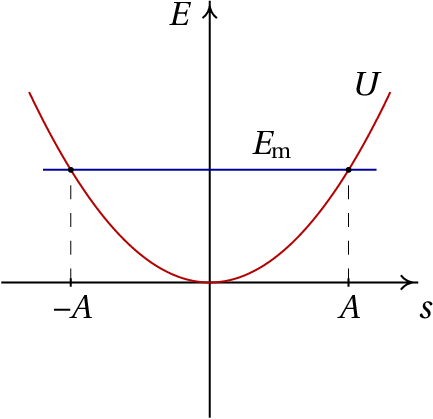Figure 6.6: Potential energy and mechanical energy of a harmonic oscillator.

The relation between amplitude and mechanical energy is obtained by substituting in equation 6.29:

(6.30)

The amplitude and the mechanical energy are not characteristic values of the oscillator, but are initial conditions that depend on how the system is set in motion. The equation of motion of the system can be obtained by applying Newton's second law, or by differentiating the expression of the mechanical energy (equation 6.29) with respect to time and solving for the tangential acceleration. The result is:

(6.31)

This expression can be used to solve the kinematic equation , with initial condition , to obtains the expression for in terms of

(6.32)

Equating this expression (in the case that is positive) to the derivative and separating variables, we obtain

(6.33)

Where time is the instant when the cart passes through the equilibrium position . Solving the integrals gives the expression for the position in terms of time

(6.34)

Where the constant , called angular frequency, is

(6.35)

and is a constant that depends on the choice of the moment when equals zero. The frequency, which is the number of oscillations per unit time, is equal to,

(6.36)

And the period of oscillation is the inverse of the frequency: .

The expression 6.34 is the solution of the differential equation . Any other system in which the second derivative of the variable equals the variable times a negative constant is consider also a simple harmonic oscillator and it has a solution similar to 6.34.

## 6.5. Kinetic energy of rotation

In a rigid body with translation motion, at every instant all parts of the body move with the same speed ; therefore, the total kinetic energy is equal to one half of the total mass times the value of the velocity squared. In the more general case of rotational and translation, to compute the total kinetic energy we must take into account that the speeds of different parts of the object are different. As shown in chapter 3, the velocity of each point in the body in terms of the angular velocity and speed of the origin, fixed point on the rigid body, is:

(6.37)

where is the position of the point relative to the origin O.

The total kinetic energy is obtained by adding the energies of all the infinitesimal parts of the rigid body with mass ,

(6.38)

The value of the velocity squared is,

(6.39)

The magnitude of is , where is the distance from the point to the axis of rotation passing through point O, parallel to . Replacing in the expression of the kinetic energy we obtain,

(6.40)

The integral in the first term is equal to the total mass . As mentioned in the section on the center of mass, the only reference frame in which the mean value of the position vector is null (equation (5.15)) is when the origin is exactly in the center of mass. Thus, if the reference point O is the center of mass, the third integral is null and we obtain

(6.41)

Where is the moment of inertia with respect to an axis passing through the center of mass, parallel to .

### Example 6.3

A sphere of mass and radius starts moving from rest at a height on an incline with an angle with the horizontal. The ball rotates on the incline without sliding. Determine the value of the angular acceleration of the sphere and the velocity of its center of mass when it reaches the end of the incline.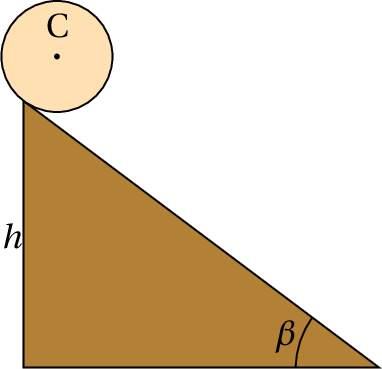Resolution. As the ball rotates without slipping, the angle of rotation is related to the position of the center of mass C, according to the expression obtained in chapter 3 for wheels rolling without slipping:

We conclude that the system has a single degree of freedom, which may be the angle that the sphere rotates from the initial instant at the top of the incline. The angular velocity is and the velocity of the center of mass is .

Choosing the Position at the top of the incline, with positive in the sense that the sphere descends and zero gravitational potential energy at , in any position the ball has descended a height , where is the angle of inclination of the incline. The total mechanical energy is,

As long as the ball rotates without slipping, the frictional force with the incline's surface is static friction, which does not do any work. Ignoring the air resistance, mechanical energy is conserved and its derivative with respect to time is zero. By replacing the expression of the moment of inertia of the sphere about its center of mass, , differentiating with respect to time and equating to zero we obtain

And the expression for the angular acceleration is,

As the sphere starts from rest, at the initial point its kinetic energy is zero and at the lowest part of the incline the kinetic energy will be equal to the change in gravitational potential energy:

(6.42)

And the velocity of the center of mass C at the end of the incline is

(6.43)

## Questions

1. The position of a particle as a function of time is given by the expression (SI units). Which of the vectors in the list is perpendicular to the trajectory of the particle at the instant  s?
2. A force with constant direction, sense and magnitude acts on a particle. The magnitude of the force is 1.6 N. What is the work done by this force when the particle moves a distance of 20 cm in a direction that makes 60° with force?
1. 0.28 J
2. 160 mJ
3. 0.68 J
4. 28 J
5. 16 J
3. In a simple harmonic oscillator formed by a body with mass hanging from a vertical spring with elastic constant , if the mass is quadrupled, which of the statements is correct?
1. Its oscillation frequency doubles.
2. Its period doubles.
3. The amplitude doubles.
4. Its mechanical energy doubles.
5. Its potential energy doubles.
4. The figure shows the plot of the potential energy of a particle in terms of the position along the trajectory, . If the particle is oscillating around the position , with mechanical energy equal to 2 J, what is the maximum value of its kinetic energy?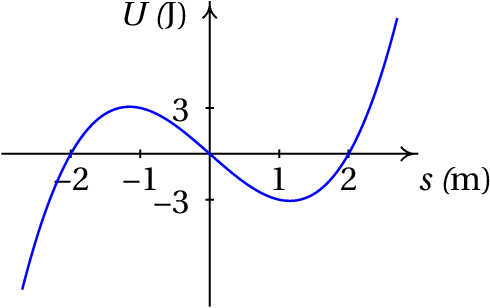1. -3 J
2. 3 J
3. 0
4. 2 J
5. 5 J
5. The figure shows the plot of the tangential component of the resultant force on a particle. How many equilibrium points are there in the region shown in the plot?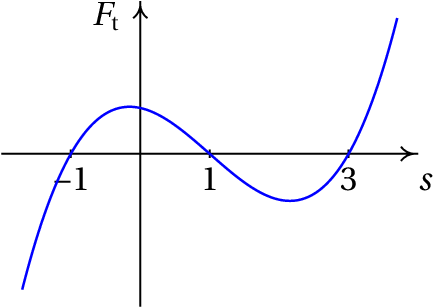1. 0
2. 1
3. 2
4. 3
5. 4

## Problems

1. Compute the line integral of the force in Example  6.2: , From the origin O to the point P in the plane , with coordinates , along the shortest arc of the circumference (center in , and radius 1) which passes through the origin and the point P.
2. The law of universal gravitation states that any celestial body of mass produces an attractive force on any other body of mass , given by the expression:
where is the universal gravitational constant, is the distance between the two bodies and is the radial unit vector, which points from the body of mass to the body of mass . (a) Determine the expression for the gravitational potential energy due to the body of mass . (b) Taking into account the result of the previous part, how can the result in equation 6.17, , be justified for the gravitational potential energy of an object on Earth?
3. In a pole vault, a 70 kg athlete uses a uniform 4.5 kg stick, 4.9 m long. The athlete's jump has three phases: first the athlete runs with its center of gravity at 1 m above the ground and with the center of gravity of the pole at 1.5 m above the ground in height, until reaching a speed of 9 m/s at the instant when he sticks the tip of the pole to the ground. In the second phase, the energy of the run is transferred to the pole, which deforms and then recovers its shape in a vertical position, raising the athlete to a height close to the height of the bar. Finally, the athlete pushes the pole towards the ground, gaining some additional energy that makes his center of gravity go higher and up to 5.8 m above the ground, enough to pass the bar at 5.6 m. Assuming there are no energy losses, compute the mechanical energy transferred to the jumper when he pushes the pole in the direction of the ground.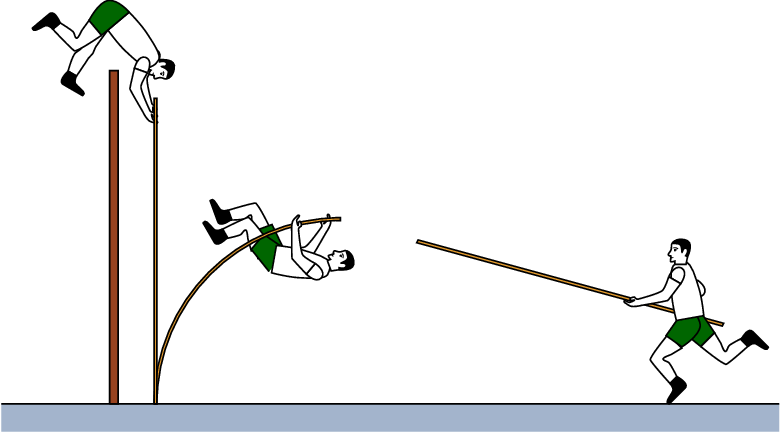4. Solve problem 7 of chapter 4 by applying the work and mechanical energy theorem. Is the force exerted by the block on the cone, while the cone penetrates the block, conservative?
5. In a system such as the one shown in figure 6.5, the cart has a mass of 450 g. The cart is moved 5 cm from the equilibrium position and released from rest, starting to oscillate with a period of 1.2 s. Determine:
1. The amplitude of the oscillations.
2. The elastic constant of the spring.
3. The maximum speed of the cart.
6. A simple pendulum is composed of a sphere of mass hanging from a very fine string with length and negligible mass. When the sphere starts to move from rest, there is a single degree of freedom, which can be the angle which the string does with the vertical. (a) Determine the expression for the mechanical energy, as a function of the angle and its derivative , using as position of zero potential energy . (b) Neglecting the air resistance, the mechanical energy remains constant and its derivative with respect to time is zero. Differentiate the expression of the mechanical energy with respect to time and equate it to zero to find the expression for in terms of the angle.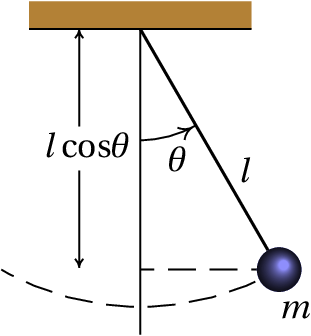7. A sphere of radius rolls without sliding, into a semicircular gutter of radius , which is on a vertical plane (see figure).
1. Show that, in terms of the derivative of the angle , the kinetic energy of the sphere is
2. Neglecting the resistance of the air, the mechanical energy is constant and its derivative with respect to time is zero. Differentiate the expression of the mechanical energy with respect to time and equate it to zero to find the expression of the angular acceleration in terms of angle.
3. Among what values ​​must the mechanical energy be so that the sphere remains oscillating within the gutter?
4. From the result of part b, determine the expression for , at the limit when the radius of the sphere is much smaller than the radius of the gutter ( ) and explain why the result is different from the result obtained for the simple pendulum in problem 6.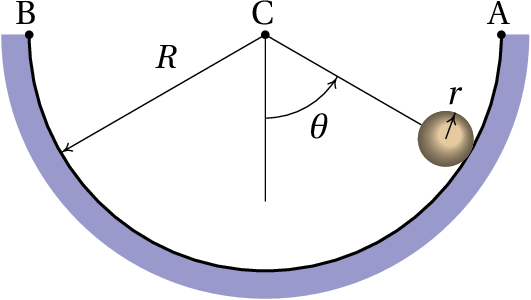8. A cylinder with mass of 80 g slides from rest at point A to point B due to a constant external force of 60 N; The normal length of the spring is 30 cm and its elastic constant is 6 N/cm. Assuming there is no friction with the fixed bar, determine the speed at which the cylinder reaches point B.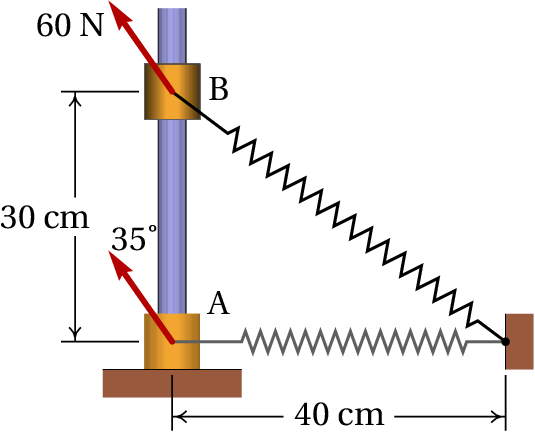9. Solve problem 8 of chapter 5 with the principle of conservation of mechanical energy.
10. A cylinder rolls down an incline of height , starting from rest, rolling without slipping. Determine the velocity of the center of mass of the cylinder when it reaches the end of the incline. Compare with the result of example 6.3 for a sphere. Which of the two bodies descends faster, the sphere or the cylinder?
11. A sphere hung with a string of length is dropped from the rest in position A, as shown in the figure. When the string reaches the vertical position, it comes into contact with a fixed nail at point B, which causes the sphere to describe an arc of radius less than . Find the minimum value that should have so that the sphere trajectory is a circle centered on B (if is not large enough, the string is no stretched while the sphere goes up and the sphere does not reach the top of the circle).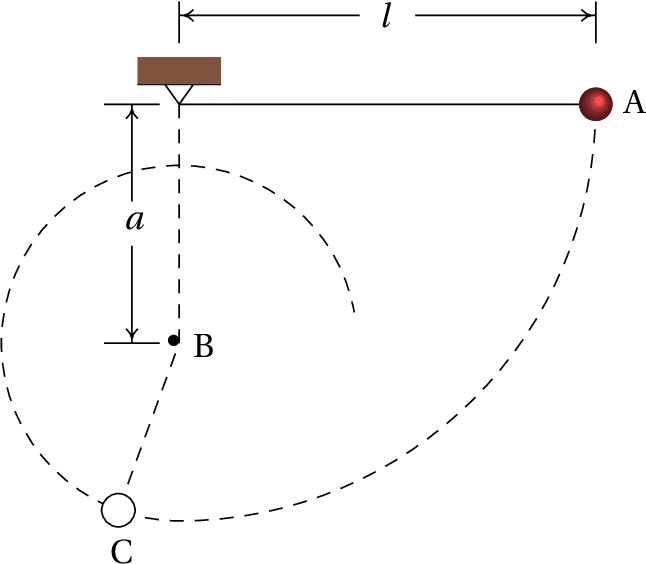12. Consider a projectile that is launched from the ground, in a room where there is vacuum, with an initial velocity making an angle with the horizontal.
1. Determine the time the projectile takes until it reaches the highest point in its trajectory, where the vertical velocity is zero, and the position at that point.
2. Based on the result of the previous part, show that the horizontal range of the projectile (horizontal distance from where it is launched to where it falls) is equal to:
(6.44)

Questions: 1. C. 2. B. 3. B. 4. E. 5. D.

Problems

1. (a)
(b) For any distance , the Taylor series of is:
The first term is a constant, which can be ignored. In the second term, if is the radius of the Earth, then is the height from the surface of the Earth and shall be equal to the constant . Ignoring the rest of the series, which for values ​​of much smaller than does not significantly change the sum of the first two terms, we obtain .
2. 317.4 J
3. 24 696 N/m2. The force of the block is not conservative, because it only acts when the cone is penetrating. If the cone rose again after penetrating the block, the block would no longer produce any force on the cone.
4. (a) 5 cm. (b) 12.34 N/m. (c) 26.2 cm/sec.
5. (a) (b)
6. (a) Note that the velocity of the center of mass of the sphere is and the condition of rolling without slipping implies that the angular velocity of the sphere is equal to that speed divided by . (b)
(c) Greater than and less than zero. If the mechanical energy is exactly equal to , the sphere does not oscillate, but remains at rest at the lowest point of the gutter. (d) The absolute value of is smaller by a factor of 5/7 because some of the gravitational potential energy is transformed into kinetic energy of rotation of the sphere. The kinetic energy of rotation is always 2/5 of the kinetic energy of translation, regardless of the value of . Therefore, at the limit also 2/7 of the gravitational energy is converted into rotational energy and only the remaining 5/7 increase .
7. 11.74 m / s.
8. 5.274 s -1
9. . The sphere descends faster than the cylinder, because it has less moment of inertia.
10. (a) ,
Wrong

This vector is not perpendicular to the trajectory, because its dot product with the velocity, , is not equal to zero.

(Click to continue)

Correct

The easiest way to find a vector perpendicular to velocity, , is to exchange the components and change the sign of one of them: , Which is 4 times the vector of letter C.

(Click to continue)

Wrong

This vector is not perpendicular to the trajectory, because its dot product with the velocity, , is not equal to zero.

(Click to continue)

Wrong

The work of a constant force is equal to the tangential component of the force times the displacement. The tangential force is equal to and not .

(Click to continue)

Correct

(Click to continue)

Wrong

The work of a constant force is equal to the tangential component of the force times the displacement.

(Click to continue)

Wrong

The force, in newton, can not be multiplied by the displacement in centimeters, because a newton equals kg·m/s2. Give the displacement in meters.

(Click to continue)

Wrong

The frequency is directly proportional to the angular frequency, which is inversely proportional to the square root of the mass. Thus, if the mass is quadrupled, the frequency decreases by half.

(Click to continue)

Wrong

The amplitude depends only on the mechanical energy, which can be altered by changing the initial conditions (initial position and velocity) independently of the mass value.

(Click to continue)

Wrong

The kinetic energy can not be negative.

(Click to continue)

Wrong

When the kinetic energy is zero, the velocity is zero. As the particle oscillates, it will have velocity different from zero at some points and, therefore, kinetic energy greater than zero.

(Click to continue)

Wrong

With this kinetic energy, the potential energy would be , Which is not the minimum value that the potential energy can have.

(Click to continue)

Correct

The maximum kinetic energy is equal to the mechanical energy, 2 J, minus the minimum potential energy, −3 J.

(Click to continue)

Wrong

The equilibrium points are the roots of the function .

(Click to continue)

Wrong

The equilibrium points are the roots of the function .

(Click to continue)

Correct

The equilibrium points are the roots of the function .

(Click to continue)